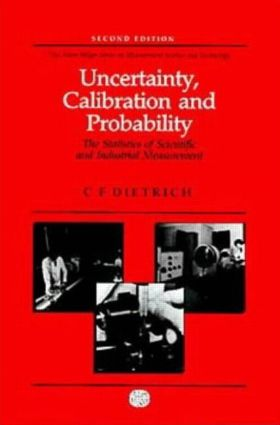Uncertainty, Calibration and Probability

The Statistics of Scientific and Industrial Measurement, 1st Edition

CRC Press

554 pages

Purchasing Options:\$ = USD
Hardback: 9780750300605
pub: 1991-01-01
SAVE ~\$84.00
\$420.00
\$336.00
x
eBook (VitalSource) : 9780203734759
pub: 2017-07-12
from \$202.50

FREE Standard Shipping!

Description

All measurements are subject to error because no quantity can be known exactly; hence, any measurement has a probability of lying within a certain range. The more precise the measurement, the smaller the range of uncertainty. Uncertainty, Calibration and Probability is a comprehensive treatment of the statistics and methods of estimating these calibration uncertainties.

The book features the general theory of uncertainty involving the combination (convolution) of non-Gaussian, student t, and Gaussian distributions; the use of rectangular distributions to represent systematic uncertainties; and measurable and nonmeasurable uncertainties that require estimation. The author also discusses sources of measurement errors and curve fitting with numerous examples of uncertainty case studies. Many useful tables and computational formulae are included as well. All formulations are discussed and demonstrated with the minimum of mathematical knowledge assumed.

This second edition offers additional examples in each chapter, and detailed additions and alterations made to the text. New chapters consist of the general theory of uncertainty and applications to industry and a new section discusses the use of orthogonal polynomials in curve fitting.

Focusing on practical problems of measurement, Uncertainty, Calibration and Probability is an invaluable reference tool for R&D laboratories in the engineering/manufacturing industries and for undergraduate and graduate students in physics, engineering, and metrology.

Uncertainties and frequency distributions

The Gaussian distribution

General distributions

Rectangular distributions

Applications

Distributions ancillary to the Gaussian

A general theory of uncertainty

The estimation of calibration uncertainties

Consistency and significance tests

Method of least squares

Theorems of Bernoulli and Stirling and the binomial, Poisson and hypergeometric distributions

Appendices

Bibliography

Index